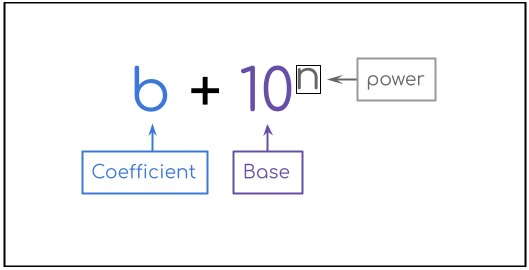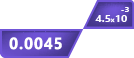# Scientific Notation Calculator

x 10^

x 10^

## Other Calculators

This write in scientific notation calculator makes math easy. Users can enter 2 numbers in scientific notation as well as integers and whole numbers.

The calculated results are represented in three formats; e, decimal, and engineering notation in this calculator. It is used for dividing, subtracting, adding, and multiplying scientific notations.

## How to use Scientific Notation Calculator?

To utilize this tool, follow these steps:

1. Enter the decimal (e.g 9.88) and exponential parts (e.g 104) of the numbers in mentioned boxes.
2. Choose the operator.
3. Click calculate.

If you want to enter whole numbers then input zero as the power of ten. For negative integers, enter (-), the subtraction symbol, with the decimal part.

## What is Scientific notation?

Scientific notation is one of the four basic formats that are used for mathematical value representation. Its general form is;The coefficient must be a number between 0 and 10. The rest of the number is represented as the power of ten.

An example of such numbers is 52341556. If you are to write this number in scientific notation, how will you do it? Firstly place the decimal point and count the digits on the right side.

“ 5.2341556 and there are 7 digits”

Now, raise 7 as the power of ten.

5.2341556  x 107”

Scientific notation has made dealing with extremely large or small numbers convenient.

To learn how to perform mathematical operations on scientific notation, keep reading.

## How to add or subtract scientific notation?

For this purpose, it is important that both numbers have the same powers of tens. The coefficients are then added or subtracted normally and the base is returned in the answer.

Example:

1. 7.9 x 106 + 1 x 106
2. 2.3 x 104 - 9.9 x 104
3. 4.5 x 103 + 2.33 x 102

Solution:

• 7.9 x 106 + 1 x 106

Powers are the same. Write the coefficients.

= 7.9 + 1

= 8.9

Now, add the common base to the answer, like this;

= 8.9 x 106

• 2.3 x 104 - 9.9 x 104

Similar to the previous question, subtract the coefficients.

= 2.3 - 9.9

= - 7.6

= -7.6 x 104

• 4.5 x 103 + 2.33 x 102

In this question, the powers are different. This means we have to change one of these in such a way that both powers become equal.

We will take the second number 2.33 x 102. Move the decimal place to the left to add 1 in the power.

= 0.233 x 103

As you can see, powers are the same now. Add the coefficients.

= 4.5 + 0.233

= 4.733 x 103

## How to divide or multiply scientific notations?

There are two rules you need to keep in mind.

1. In division, powers are subtracted.
2. In multiplication, powers are added.

It will be more clear through examples.

Example:

Divide 4.5 x 108 by 2.13 x 103. What will be the answer if you multiply the same numbers.

Solution:

Step 1: Separate the coefficients and the bases.

= (4.5 / 2.13) + (108/ 103)

Step 2: Divide the coefficients.

= 2.11 + (108/ 103)

Step 3: Subtract the power of the denominator from the power of the numerator.

= 2.11 + (108-3)

= 2.11 + 105

This was for the division part. For multiplication, see the solution below.

Solution:

Step 1: Separate the coefficients and bases.

= (4.5 x 2.13) + (108 x 103)

Step 2: Divide the coefficients.

= 9.6 + (108 x 103)

Step 3: Add the powers.

= 9.6 + (108+3)

= 9.6 + 1011